# extracting features from images of the sun¶

In this notebook, we will extract features from images taken by the Atmospheric Imaging Assembly (AIA) instrument on NASA's Solar Dynamics Observatory (SDO) satellite by co-aligning them with the Helioseismic and Magnetic Imager (HMI) instrument's Space-weather HMI Active Region Patch (SHARP) data. The AIA instrument takes images of the Sun's atmosphere, or corona, in ultraviolet light; the HMI instrument takes images of the Sun's photosphere, or surface, in optical light. The HMI SHARP data encapsulates automatically-identified solar active regions. It's easy to use the SHARP data as a stencil to cut out similarly active areas in the corona.

During solar flares, the Sun's corona gets particularly bright. Here's a video of one of the largest flares that erupted over the last five years:

In :
from IPython.display import YouTubeVideo

Out:

We still do not fully understand why a solar flare releases so much energy so fast, and what, exactly, the magnetic topology of the corona looks like during a flare. One commonly, though not universally, accepted theory is that ropes of magnetic field, called flux ropes, hang out in the chromosphere, or the layer between the solar surface and corona. These ropes twist up and release energy during a flare. If that's true, we should look at the chromosphere for clues. Let's grab an image showing a patch of the chromosphere where the flare takes place. First, let's import some modules:

In :
import json, urllib, numpy as np, matplotlib.pylab as plt, matplotlib.ticker as mtick, requests
import sunpy.map
import drms
from astropy.io import fits
from sunpy.cm import color_tables as ct
import sunpy.wcs as wcs
from datetime import datetime as dt_obj
import matplotlib.dates as mdates
import matplotlib.colors as mcol
import matplotlib.patches as ptc
from matplotlib.dates import *
import math


Now, let's query the JSOC database, where all the SDO data are stored, using the JSON API to retrieve both keywords and the location of the AIA chromospheric image files. The first step is to establish a connection to JSOC. This can be done with the drms.Client() class. Here is more information on how to download SDO data using the drms module.

In :
import drms
c = drms.Client()


The astropy library's fits.open() call downloads the data.

In :
keys_aia, segments = c.query('aia.lev1[2012.03.06_23:29:06_TAI/12s][?WAVELNTH=1600?]', key='T_REC,CROTA2,CDELT1,CDELT2,CRPIX1,CRPIX2,CRVAL1,CRVAL2', seg='image_lev1')
url_aia = 'http://jsoc.stanford.edu' + segments.image_lev1   # add the jsoc.stanford.edu suffix to the segment name


We queried for a few keywords. We'll need these keywords later to coalign these data with others. For the AIA data, this is what they mean:

• T_REC indicates the time the image was taken (technically the mid-point of the integration time),
• CROTA2 indicates the rotation of the camera with respect to the fixed Sun in degrees,
• CDELT1n indicates the platescale of the instrument in arseconds per pixel,
• CRPIXn indicates the the location of the center of the Sun on the CCD in pixels, and
• CRVALn indicates the same thing as CRPIXn, but in arcseconds.

Here's an image of the chromosphere for the same flare as the YouTube video above. The SunPy library's peek() function allows us to look at the data in the standard AIA color tables without much effort at all:

In :
sunpy.map.Map(url_aia).peek()
plt.show()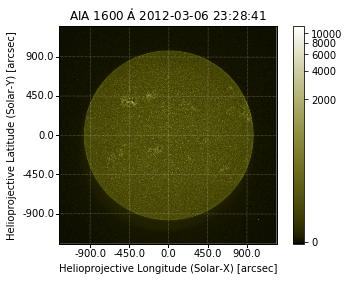The Helioseismic and Magnetic Imager (HMI), another instrument aboard SDO, takes images of the solar surface, or photosphere. Here's an image of the photosphere taken at the same time as the chromospheric image above. You can see that it is rotated 180 degrees with respect to the AIA image, which is why it is important for us to take the CROTA2 value for each instrument into consideration when doing any array manipulation.

In :
keys_hmi, segments = c.query('hmi.M_720s[2012.03.06_23:29:06_TAI]', key=drms.const.all, seg='magnetogram')
url_hmi = 'http://jsoc.stanford.edu' + segments.magnetogram   # add the jsoc.stanford.edu suffix to the segment name

In :
header = dict(keys_hmi.iloc)

In :
# Add a DATE-OBS keyword which seems to be required by sunpy.map.Map.

# Add HGLN_OBS keyword to avoid a warning in sunpy.map.Map.

In :
sunpy.map.Map(photosphere_full_image, header).peek()
plt.show()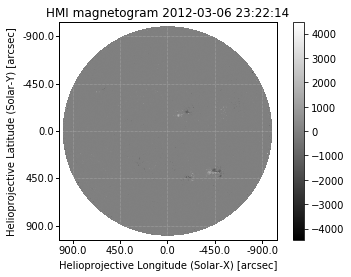The images above are 4096x4096 pixels, but binned down significantly. Let's zoom in on the active region. There's a particular type of SDO data called HMI Active Region Patches, or HARPs. These are bitmaps that identify automatically-detected active regions. Specifically, the bitmap array assigns a value to each pixel inside the bounding box, depending on whether it i) resides inside or outside the active region, and ii) corresponds to weak or strong line-of-sight magnetic field. This is what these patches look like for our selected T_REC:

In :
from IPython.display import Image
Image("http://jsoc.stanford.edu/doc/data/hmi/harp/harp_definitive/2012/03/06/harp.2012.03.06_23:00:00_TAI.png")

Out: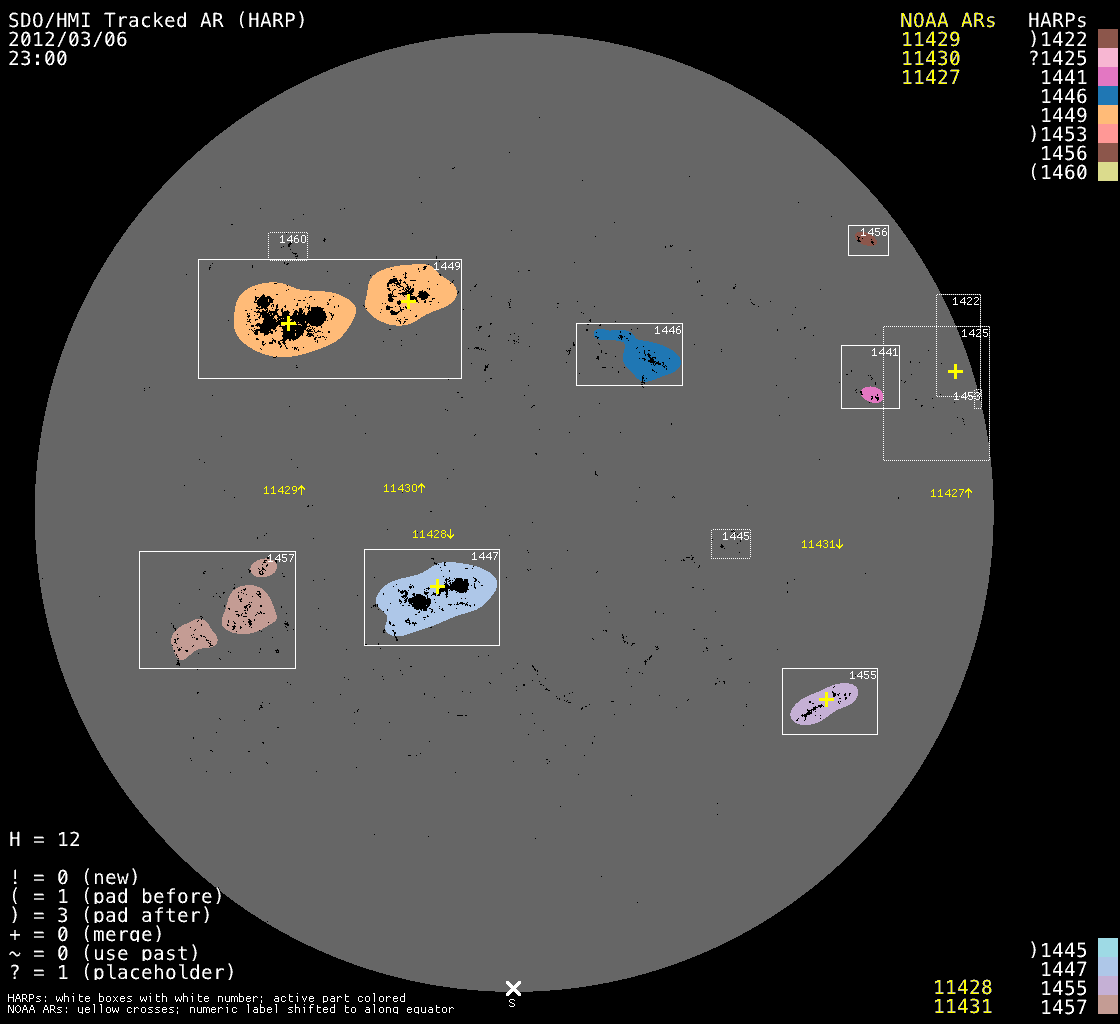We use the HARP bounding box as a stencil to cut out patches of data from full-disk images. The full-disk SDO data use the standard World Coordinate System for solar images (Thompson, 2006). The patch data, which we call Space-weather HMI Active Region Patches (or SHARPs), are available in either of two coordinate systems: one is effectively cut out directly from corrected full-disk images, which are in Helioprojective Cartesian CCD image coordinates, and the other is remapped from CCD coordinates to a Carrington Heliographic Cylindrical Equal-Area (CEA) projection centered on the patch.

Now, we'll identify which AIA pixels are included inside each of these SHARP bounding boxes. First, let's go through an example for HARP number 1449 for our selected T_REC for the CCD case.

### identifying which AIA pixels are included in the CCD-coordinate SHARP bounding box¶

First, we query for CCD-coordinate SHARP data:

In :
keys_ccd, segments = c.query('hmi.sharp_720s[2012.03.06_23:29:06_TAI]', key=drms.const.all, seg='magnetogram')
url_ccd = 'http://jsoc.stanford.edu' + segments.magnetogram   # add the jsoc.stanford.edu suffix to the segment name
XDIM_CCD = photosphere_image.data.shape
YDIM_CCD = photosphere_image.data.shape


• CRPIXn indicates the location, in pixels, of disk center with respect to the lower left-hand corner of the patch,
• CROTA2 indicates the rotation of the camera with respect to the fixed Sun in degrees,
• CDELTn indicates the platescale of the instrument, in arcseconds per pixel, and
• XDIM and YDIM give the x and y-dimensions of the image, respectively, in pixels.

Then we must make sure that the angle between the HMI camera and the fixed Sun is approximately zero; otherwise, we'll rotate the image to ensure this is so.

In :
if (keys_ccd.CROTA2 > 5.0):
print("The HMI camera rotation angle is",keys_ccd.CROTA2,". Rotating HMI images.")
photosphere_full_image = np.rot90(photosphere_full_image,2)
photosphere_image.data = np.rot90(photosphere_image.data,2)

The HMI camera rotation angle is 180.082581 . Rotating HMI images.


Now we can plot HARP number 1449 at this T_REC in CCD coordinates (using the SunPy color table for SDO images):

In :
hmimag = plt.get_cmap('hmimag')
plt.imshow(photosphere_image.data,cmap=hmimag,origin='lower')
print('The dimensions of this image are',photosphere_image.data.shape,'by',photosphere_image.data.shape,'.')

The dimensions of this image are 480 by 1056 .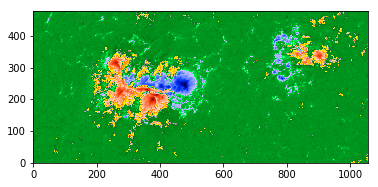We can identify this area on the full-disk photospheric image in CCD coordinates for a sanity check:

In :
fig, ax = plt.subplots(1,1)
plt.imshow(photosphere_full_image,cmap=hmimag,origin='lower',vmax=300,vmin=-300,extent=[0,4096,0,4096])
y1 = (2048. + keys_ccd.CRPIX2 - YDIM_CCD)
y2 = (2048. + keys_ccd.CRPIX2)
x1 = (2048. + keys_ccd.CRPIX1 - XDIM_CCD)
x2 = (2048. + keys_ccd.CRPIX1)

# draw a box using matplotlib.patches.Rectangle
ax.add_patch(ptc.Rectangle((x1, y1), XDIM_CCD, YDIM_CCD, hatch='///////', fill=False, snap=False))

# plot a green dot at the lower left-hand corner
plt.plot(x1, y1, 'g.', markersize=10.0)

# plot a red dot at the upper right-hand corner
plt.plot(x2, y2, 'r.', markersize=10.0)
fig.set_size_inches(6,6)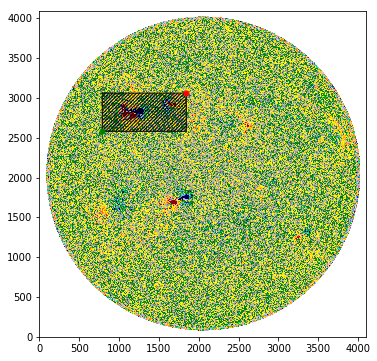If we are interested in cutting a SHARP-sized box out of AIA data, the first thing we can do is compute the ratio of the platescales. Since HMI and AIA are not observing in the same wavelength, the radius of the solar disk is different in both images and, as a result, the platescale is too:

In :
ratio = (keys_ccd.CDELT1)/(keys_aia.CDELT1)
print("The ratio of the HMI:AIA platescales is",ratio,".")

The ratio of the HMI:AIA platescales is 0.8275571776235573 .


Now we can cut the same sized array out of the AIA data. We first have to use the astropy package to fix the FITS header as some of the AIA keywords have non-standard values. Then we must again make sure that the angle between the camera and the fixed Sun is approximately zero; otherwise, we'll rotate the image to ensure this is so.

In :
chromosphere_image.verify("fix")
if (keys_aia.CROTA2 > 5.0):
print("The AIA camera rotation angle is",keys_aia.CROTA2,". Rotating AIA image.")
chromosphere_image.data = np.rot90(chromosphere_image.data,2)

In :
y1 = int(np.rint(2048. + keys_ccd.CRPIX2*(ratio) - YDIM_CCD*(ratio)))
y2 = int(np.rint(2048. + keys_ccd.CRPIX2*(ratio)))
x1 = int(np.rint(2048. + keys_ccd.CRPIX1*(ratio) - XDIM_CCD*(ratio)))
x2 = int(np.rint(2048. + keys_ccd.CRPIX1*(ratio)))

In :
subdata = chromosphere_image.data[y1:y2, x1:x2]


Now we can look at the patch of chromospheric data taken by the AIA instrument. Though it is not easy to see, the twisted S-shape feature may be the signature of a flux rope.

In :
sdoaia1600 = plt.get_cmap('sdoaia1600')
plt.imshow(subdata,cmap=sdoaia1600,origin='lower',vmin=0,vmax=400)
print('The dimensions of this image are',subdata.shape,'by',subdata.shape,'.')

The dimensions of this image are 397 by 874 .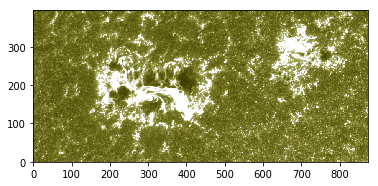You can see the chromospheric patch is a bit smaller, in both latitudinal and longitudinal extent, from the photospheric data taken by the HMI instrument. This is, again, exactly what we expect since the solar radius is a bit smaller in the ultraviolet wavelengths taken by AIA versus the optical wavelengths observed by HMI.

### identifying which AIA pixels are included in the CEA-coordinate SHARP bounding box¶

Now we can go through the same exercise for CEA-coordinate SHARP data. The CEA data are useful for two reasons:

1. Each pixel is of an equal area, so derivatives or other such numerical computations are straightforward, and
2. The space-weather keywords included in the SHARP metadata, which characterize the photospheric vector magnetic field, are computed using the CEA data; as such, computing a feature using a CEA-sized cutout of the AIA data allows for easy comparison between the space-weather keywords and any feature derived from the AIA data.
In :
keys_cea, segments = c.query('hmi.sharp_cea_720s[2012.03.06_23:29:06_TAI]', key=drms.const.all, seg='magnetogram')
url_cea = 'http://jsoc.stanford.edu' + segments.magnetogram   # add the jsoc.stanford.edu suffix to the segment name
XDIM_CEA = cea_image.data.shape
YDIM_CEA = cea_image.data.shape


This is what the keywords mean in the Carrington Heliographic Cylindrical Equal-Area coordinate system:

• CRLT_OBS indicates the Carrington latitude of the observer in degrees,
• CDELTn indicates the platescale of the image in degrees per pixel, which, per the definition of equal-area, is always constant (0.03 degrees per pixel),
• CRVALn indicates the longitude and latitude at the center of the patch, in degrees,
• CRLN_OBS indicates the Carrington longitude of the observer in degrees, and
• XDIM and YDIM give the x and y-dimensions of the image, respectively, in pixels.
In :
plt.imshow(cea_image.data,cmap=hmimag,origin='lower')
print('The dimensions of this CEA-projected image are',cea_image.data.shape,'by',cea_image.data.shape,'.')

The dimensions of this CEA-projected image are 460 by 1167 .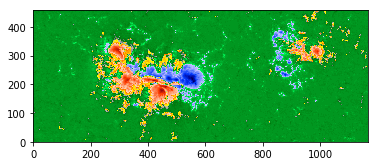To map the CEA data to the AIA data, we follow the steps outlined in Sun et al. (2014). We first convert the latitude and longitude at the center of the patch from the Carrington Heliographic coordinate system, which has units of degrees, to Helioprojective Cartesian coordinates, which has units of arcseconds. To do this, we use SunPy's WCS module. After we know the center of the patch in units of arcseconds, we can use the platescale to convert it to units of AIA pixels. Let's plot three points, indicating the center, upper right-hand corner, and lower left-hand corner of the CEA bounding box on the AIA data:

In :
fig, ax = plt.subplots(1,1)
plt.imshow(chromosphere_image.data,origin='lower',cmap=sdoaia1600,vmin=0,vmax=400,extent=[0,4096,0,4096])
#plt.colorbar()

#  plot a blue dot at the center of the CEA box on the AIA image:

# convert from Carrington Heliographic to Helioprojective Cartesian coordinates
# CRVALn_CEA is the center of the CEA box in Carrington Heliographic coordinates (degrees)
# CRLT_OBS_CEA is the Heliographic latitude of the observer in (degrees)
# CRLN_OBS_CEA is the Carrington longitude of the observer in (degrees)
# thus HPC_out is the center of the CEA box in Helioprojective-Cartesian coordinates (arcsec)
HPC_out = wcs.convert_hg_hpc(keys_cea.CRVAL1, keys_cea.CRVAL2, b0_deg=keys_cea.CRLT_OBS, l0_deg=keys_cea.CRLN_OBS)

# CRPIXn_AIA is the center of the AIA image in AIA pixels
# CDELT1_AIA is the platescale of the AIA data in arcsec/AIA pixel
# thus (xc,yc) is the center of the CEA box in AIA pixels
xc = ((HPC_out)/keys_aia.CDELT1) + keys_aia.CRPIX1
yc = ((HPC_out)/keys_aia.CDELT1) + keys_aia.CRPIX2
plt.plot(xc, yc, 'b.', markersize=10.0)

#  plot a green dot at the lower left-hand corner of the CEA box on the AIA image:
# mDIM_CEA are the dimensions of the CEA box in HMI pixels
# CDELT1_CEA is the platescale of the CEA image in degrees/CEA pixel
# thus (x1,y1) is the lower left-hand corner of the CEA image in Carrington Heliographic coordinates (degrees)
x1 = keys_cea.CRVAL1 - 0.5*XDIM_CEA*keys_cea.CDELT1
y1 = keys_cea.CRVAL2 - 0.5*YDIM_CEA*keys_cea.CDELT1

# convert from Carrington Heliographic to Helioprojective Cartesian coordinates
HPC_out = wcs.convert_hg_hpc(x1, y1, b0_deg=keys_cea.CRLT_OBS, l0_deg=keys_cea.CRLN_OBS)
x1 = ((HPC_out)/keys_aia.CDELT1) + keys_aia.CRPIX1
y1 = ((HPC_out)/keys_aia.CDELT1) + keys_aia.CRPIX2
plt.plot(x1, y1, 'g.', markersize=10.0)

#  plot a red dot at the upper right-hand corner of the CEA box on the AIA image:
# mDIM_CEA are the dimensions of the CEA box in HMI pixels
# CDELT1_CEA is the platescale of the CEA image in degrees/CEA pixel
# thus (x1,y1) is the lower left-hand corner of the CEA image in Carrington Heliographic coordinates (degrees)
x2 = keys_cea.CRVAL1 - 0.5*XDIM_CEA*keys_cea.CDELT1 + XDIM_CEA*keys_cea.CDELT1
y2 = keys_cea.CRVAL2 - 0.5*YDIM_CEA*keys_cea.CDELT1 + YDIM_CEA*keys_cea.CDELT1

# convert from Carrington Heliographic to Helioprojective Cartesian coordinates
HPC_out = wcs.convert_hg_hpc(x2, y2, b0_deg=keys_cea.CRLT_OBS, l0_deg=keys_cea.CRLN_OBS)
x2 = ((HPC_out)/keys_aia.CDELT1) + keys_aia.CRPIX1
y2 = ((HPC_out)/keys_aia.CDELT1) + keys_aia.CRPIX2
plt.plot(x2, y2, 'r.', markersize=10.0)

fig.set_size_inches(8,8)